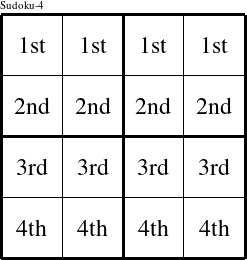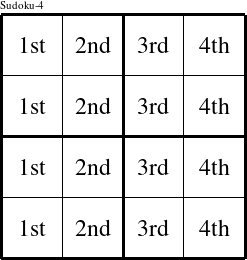#### Printable version

Detailed instructions for working a group puzzle of type 'Rita'

This is a signature puzzle named 'Rita'

The 4 elements in each group of this type of puzzle are: R, I, T, and A.

Every group in the puzzle must contain exactly this set of elements.

####In a Rita puzzle, each row is a group numbered as shown in the figure above.

####In a Rita puzzle, each column is a group numbered as shown in the figure above.

####In a Rita puzzle, each 2x2 square is a group numbered as shown in the figure above.